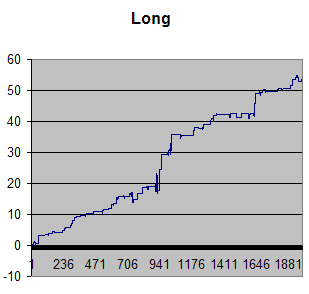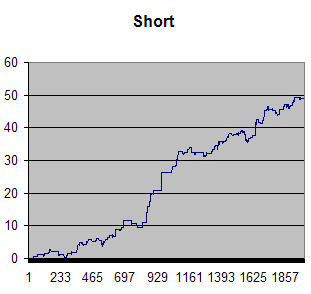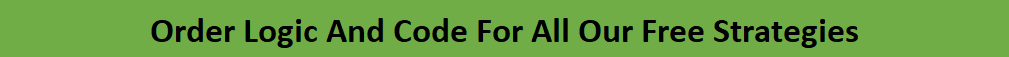# When XLP Diverges From Recent High And Low: A Mean Reversion Trading Strategy

Last Updated on June 19, 2022 by Quantified Trading

A while back I wrote about a day trading strategy in SPY. This one works pretty well on XLP as well, with some modifications:

## The high and low divergence mean reversion trading strategy

The strategy is like this in plain English:

1. Calculate a 25 day average of the (High minus Low – (H-L)). That is the “ATR”.
2. Calculate the Low of the last 10 days.
3. Calculate the (C-L)/(H-L) ratio every day (IBS).
4. Calculate a band 2.5 times above the 10 day low using the average from point number 1 (ATR).
5. If XLP closes above the band in number 4, and point 3 has a higher value than 0.8, then go short at the close.
6. Exit on tomorrow’s close.

Vice versa for long except the value in number 3 must be below 0.33.

Test period from 2005 until 2013. Here are the results:

Long:

 Total % #Fills #Wins Average 53.57363 161 106 0.333

Profit curve:Short:

 Total P/L #Fills #Wins Average 49.03834 237 141 0.207

Equity curve:By the way, this strategy has been horrible in 2013 on the short side (live trading).

——————–

If you would like to have the Amibroker or Tradestation code for this strategy plus 70+ other free trading strategies published on this website, please click on this link:## Similar Posts

•Jack Weinberg says:

Question: In your formula point #4:
Calculate a band 2.5 times above the 10 day low using the average from point number 1 (ATR).

Is the actual calculation of the Upper Band as follows:
(pseudo code)
UpperBand = 2.5 * LowestLow (past 10 days) + Moving Average(H+L, 25)

I am having some difficulty in reproducing your results on XLP, and it would be greatly appreciated if you could just clarify that one point.

•Oddmund Grotte says: